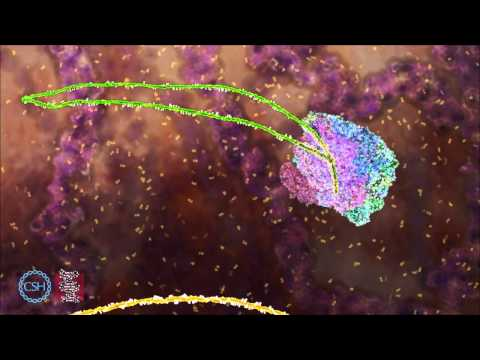Skip to content

# Splice Site Classification

As an example of the PyTorch integration this example will use the UCI Splice-junction Gene Sequences dataset on UCI.

In short, splice sites are locations where the introns and exons connect on pre-mRNA. It's important to understand where these are because the process of splicing removes introns so the exons from the pre-mRNA form mRNA. And mRNA becomes proteins.

Put another way, understanding where the splice sites are helps determine which parts of DNA become proteins.

This is a great 1m37s video from the DNA Learning Center for more context.From an ML perspective, this is a classification where the sequence is a feature and there is a label, one of:

• Intron to exon (IE) -- Does this sequence contain an intron to exon junction.
• Exon to intron (EI) -- Does this sequence contain an exon to intron junction.
• Neither (N) -- Does this sequence contain neither.

## Loading Data

To help load data GCGC contains a `FileMetaDataField` class that can help turn files into labels.

In this example, the easiest path is to use the `SPLICE_DATA_PATH` constant, which will point to the path containing the files EI.fasta, IE.fasta, and N.fasta. The labels for the dataset will become those files names due to the field.

```from gcgc.fields.categorical_field import FileMetaDataField
from gcgc.data import SPLICE_DATA_PATH

files = list(SPLICE_DATA_PATH.glob("*.fasta"))
# [PosixPath('/home/tshauck/gcgc/gcgc/data/splice/EI.fasta'),
#  PosixPath('/home/tshauck/gcgc/gcgc/data/splice/IE.fasta'),
#  PosixPath('/home/tshauck/gcgc/gcgc/data/splice/N.fasta')]
# Each class is a file.

file_feature = FileMetaDataField.from_paths("splice_site", files)
```

`file_feature` is one possible features someone might want to model -- in this case the splice classification. A `SequenceParser` object can be holds possible features, and in this case the `TorchSequenceParser` will go one step further by converting the outputs of the SequenceParser into native torch objects. See the documentation on sequence parsers for more info.

```from gcgc.ml.pytorch_utils.parser import TorchSequenceParser

parser = TorchSequenceParser(file_features=[file_feature])
```

The `SequenceParser` can also configure how GCGC will deal with sequences of difference length.

There is one more preparatory step needed before creating the PyTorch compatible `Dataset`, and that is to make a GCGC Alphabet. These Alphabet's are subclasses of BioPython Alphabet's class that support padding, tokenizing and other options relevant for ML applications.

In this case, `IUPACAmbiguousDNAEncoding` is relevant for DNA with the standard letters plus extra to deal with ambiguous bases. One example of an ambiguous base letter is that "R" is Purine, which can be either Adenine ("A") or Guanine ("G").

```from gcgc.alphabet import IUPACAmbiguousDNAEncoding

alphabet = IUPACAmbiguousDNAEncoding()
```

Those ingredients set the stage to create the `GenomicDataset` object

```from gcgc.ml.pytorch_utils.data import GenomicDataset

dataset = GenomicDataset.from_paths(files, parser, alphabet=alphabet)
```

Given a set of files, `from_paths` is the best option for generating a `GenomicDataset`. GCGC uses BioPython's indexing to work with large files typically found from sequencing samples, and `from_paths` will generate the indexes for you with the proper alphabet.

## Training

Given the dataset, it's now possible to use PyTorch's built-in `DataLoader` object which helps with shuffling, batching, and other common data utilities.

```from torch.utils.data import DataLoader

data_loader = DataLoader(dataset, batch_size=128, shuffle=True)
```

With the data_loader configured we'll create a simple PyTorch model that applies a single 1d convolution layer then a series of dense layers. The output corresponds to the three classes: EI, IE, N.

```import torch.nn as nn
import torch.nn.functional as F

class SplicePrediction(nn.Module):
def __init__(self, vocab_size, num_labels, embed_size=2, num_filters=2, kernel_size=1):
super().__init__()

self.embed = nn.Embedding(vocab_size, embed_size)
self.conv = nn.Conv1d(embed_size, num_filters, kernel_size)

self.fc1 = nn.Linear(124, 100)
self.fc2 = nn.Linear(100, 50)
self.fc3 = nn.Linear(50, num_labels)

def forward(self, inputs):
embed = self.embed(inputs).permute(0, 2, 1)

x = self.conv(embed)
x = x.view(x.size(), -1)
x = self.fc1(F.relu(x))
x = self.fc2(F.relu(x))
x = self.fc3(x)

return x
```

Now, it's a matter of training the model by passing it data from the loader. Importantly, the object iterated through via `data_loader` has access to a set of keys that are PyTorch tensor object.

`seq_tensor` is always present, and represents the input sequence represented as a PyTorch `LongTensor`. Also in the example below notice that the `splice_site` key is present which was the name of the `FileMetaDataField` used earlier and represents which class the sequences is a member of.

```import torch

vocab_size = len(alphabet)
num_labels = len(files)
model = SplicePrediction(vocab_size, num_labels)

optimizer = torch.optim.Adam(model.parameters(), lr=0.001)
criterion = torch.nn.CrossEntropyLoss(size_average=False)

model.train()

for i in range(10):  # 10 epochs
for batch in data_loader:  # Created from the GenomicDataset
sequences = batch["seq_tensor"].to("cpu")
target = batch["splice_site"].to("cpu")

optimizer.zero_grad()
output = model(sequences)
loss = criterion(output, target)
loss.backward()
optimizer.step()
```

Because this isn't an example of PyTorch generally, this example doesn't include important considerations like cross-validation, metrics, etc, but hopefully it's clearer how to use GCGC in conjunction with PyTorch.